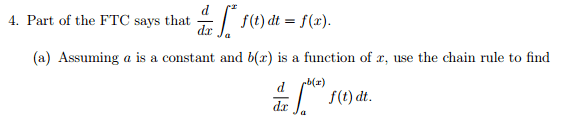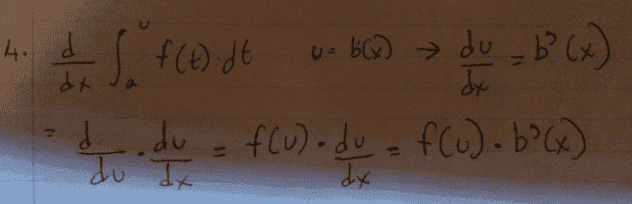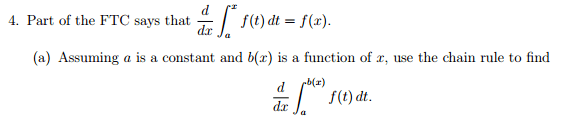# Fundamental Theorem of Calculus

planauts

## Homework Statement## The Attempt at a Solution

I know this is not right.Could someone help me out here?
Thanks

Staff Emeritus
Homework Helper
Gold Member

## Homework Statement## The Attempt at a Solution

I know this is not right.Could someone help me out here?
Thanks
Since u = b(x) your final answer is equivalent to $f(b(x))\cdot b'(x)\ .$